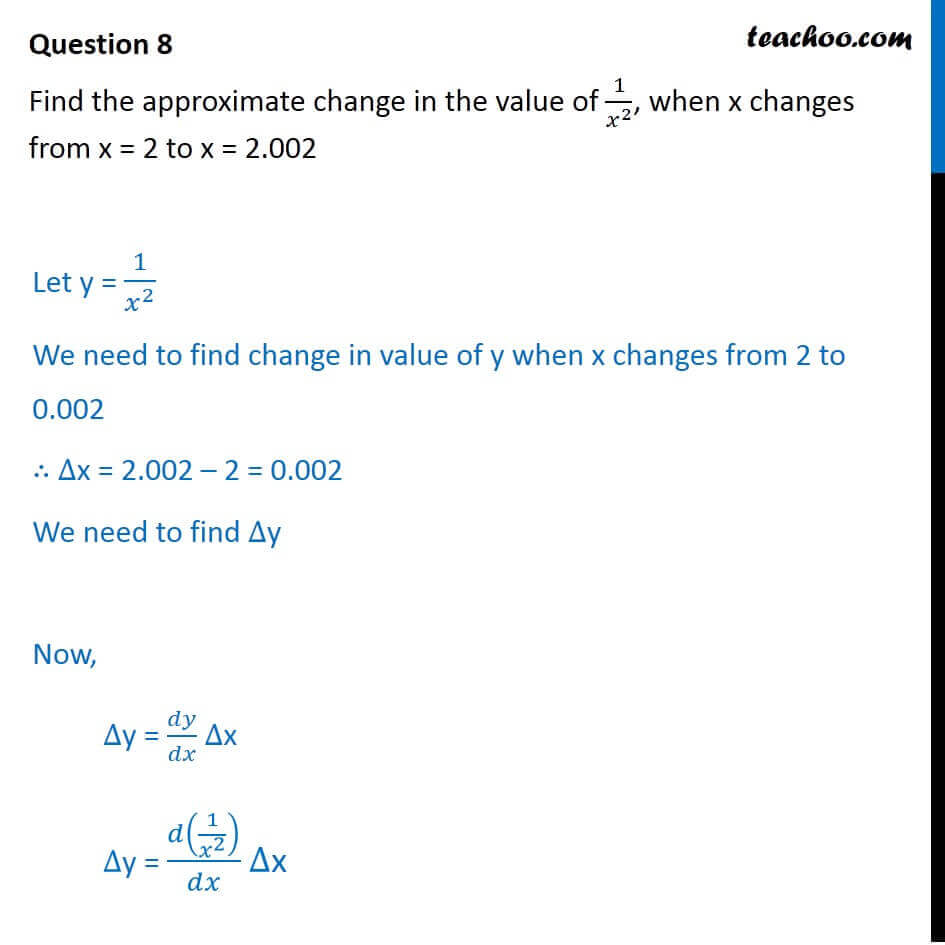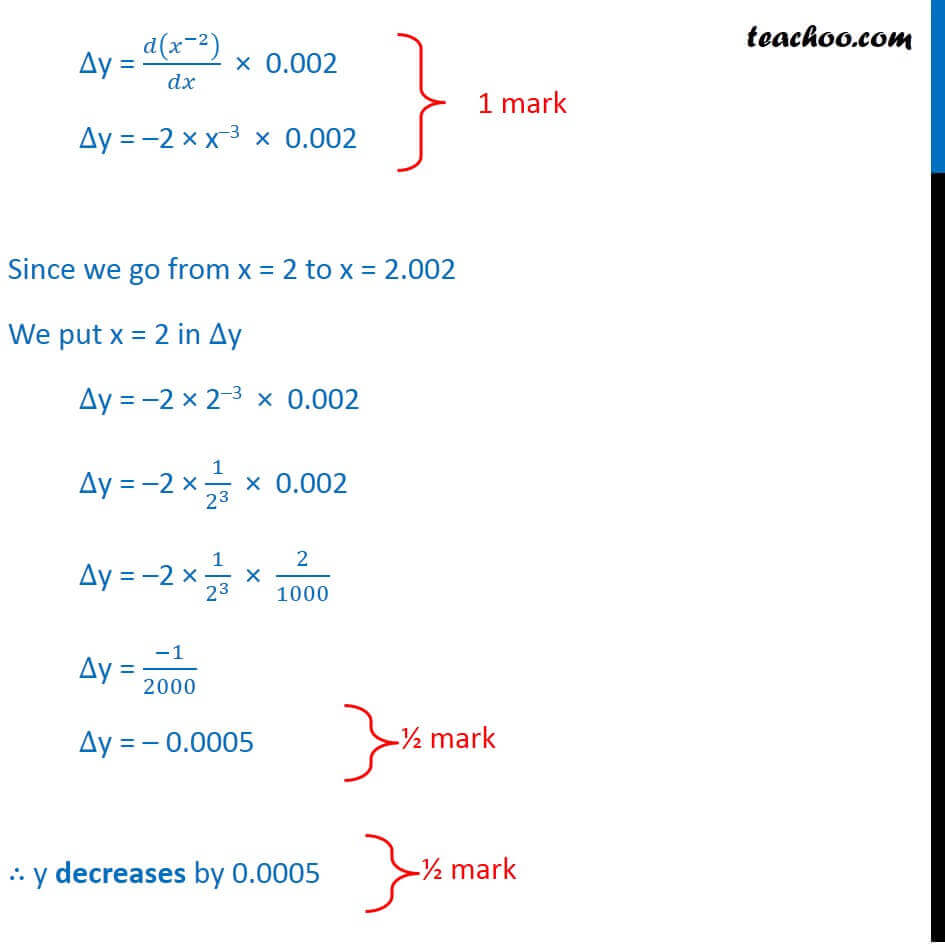CBSE Class 12 Sample Paper for 2018 Boards

Class 12
Solutions of Sample Papers and Past Year Papers - for Class 12 Boards

### Find the approximate change in the value of 1/x 2 , when x changes from x = 2 to x = 2.002

This is a question of CBSE Sample Paper - Class 12 - 2017/18.Get live Maths 1-on-1 Classs - Class 6 to 12

### Transcript

Question 8 Find the approximate change in the value of 1/𝑥^2 , when x changes from x = 2 to x = 2.002 Let y = 1/𝑥^2 We need to find change in value of y when x changes from 2 to 0.002 ∴ ∆x = 2.002 – 2 = 0.002 We need to find ∆y Now, ∆y = 𝑑𝑦/𝑑𝑥 ∆x ∆y = 𝑑(1/𝑥^2 )/𝑑𝑥 ∆x ∆y = 𝑑(𝑥^(−2) )/𝑑𝑥 × 0.002 ∆y = –2 × x–3 × 0.002 Since we go from x = 2 to x = 2.002 We put x = 2 in ∆y ∆y = –2 × 2–3 × 0.002 ∆y = –2 × 1/2^3 × 0.002 ∆y = –2 × 1/2^3 × 2/1000 ∆y = (−1)/2000 ∆y = – 0.0005 ∴ y decreases by 0.0005